```

```
Wholeness and the Implicate Order
```
```
The Crystallization Process and the Implicate Order
(Part Six)

```

```
We  will now investigate the generation of the Plane Group C2mm, i.e. the generation of its group elements.
```
```
The Plane Group C2mm

Placing motifs with 2mm symmetry in a centered rectangular net (= centered rectangular 2-D lattice) yields the Plane Group C2mm. See Figure 1.Figure 1.  Placing motifs with point symmetry 2mm in a centered rectangular net, yields a periodic array of motifs representing Plane Group C2mm.
As in all cases, the pattern must be imagined to be extended indefinitely across the two-dimensional plane.

```
```Figure 2.  A unit mesh choice is given (yellow). This unit mesh has point symmetry 2mm, and is centered.

```
```
If we eliminate all translations from the pattern representing Plane Group C2mm, then we end up with a figure that has point symmetry 2mm. So the Point Group associated with that Plane Group is indeed 2mm. See Figure 3.Figure 3.  The translation-free residue of the pattern representing Plane Group C2mm. The resulting figure represents Point Group 2mm.

```
```
The unit mesh, as given in Figure 2, contains two motifs s.str. (1 + 1/4 + 1/4 + 1/4 + 1/4). The whole unit mesh with its content can be considered as the motif s.l. that tiles the 2-D plane completely.

Patterns representing the Plane Group C2mm have 2-fold rotation axes, mirror lines and glide lines. See Figures 4---7.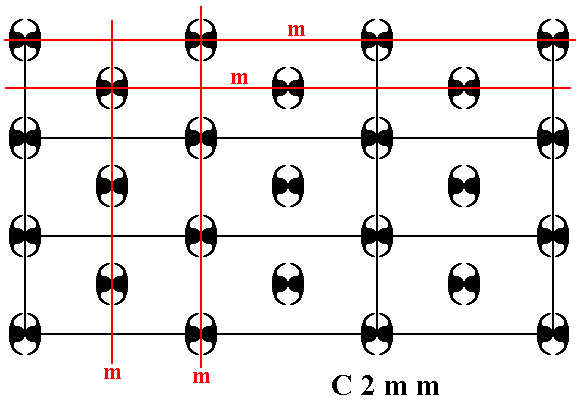Figure 4.  A pattern representing Plane Group C2mm has mirror lines in the x and y directions. Some of them are indicated (m).

```
```Figure 5.  A pattern representing Plane Group C2mm has 2-fold rotation axes perpendicular to the plane of the drawing. Some of them are indicated (red dots).

```
```Figure 6.  A pattern representing Plane Group C2mm has glide lines.
One of them is indicated
(g).

```
```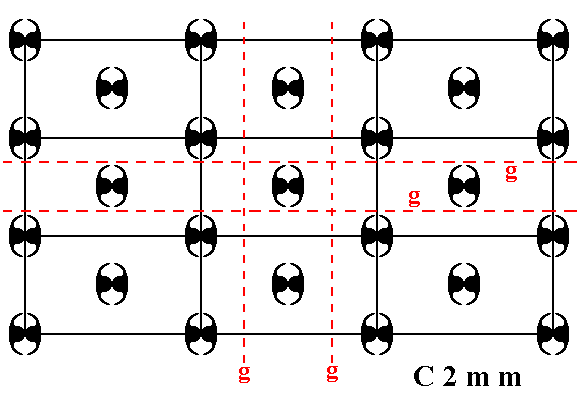Figure 7.  A pattern representing Plane Group C2mm has glide lines. Some of them are indicated (g).

```
```
The total symmetry content of any pattern representing Plane Group C2mm is given in the next Figure.Figure 8.  Total symmetry content of Plane Group C2mm.
2-fold rotation axes perpendicular to the plane of the drawing are indicated by small solid red ellipses.
Mirror lines are indicated by solid lines (black, and red).
Glide lines are indicated by red dashed lines.

```

```
The generation of the group elements of the Plane Group C2mm

The next Figure shows three generators that can generate the whole pattern :
The element  a  that results from the element  1  (chosen to represent the identity element) by a reflection in the line  a .
The element  b  that results from the element  1  by a reflection in the line  b .
The element  s  that results from the element  1  by a half-turn about the point  S .
These three generators together can produce all other group elements (each such element may be represented by a motif unit).Figure 9.  The three chosen generators for the  C2mm  pattern.

```
```Figure 10.  The  C2mm  pattern can be generated by the elements  a, b  and  s .
The right part of the pattern can be reached as follows :
From the generated element
absabsba  (lower right) we can generate a new element by rotating it 1800 about the point  S .  This new element is situated to the left of the part of the pattern depicted (i.e. it lies outside the depicted part of the pattern). When we now reflect this new element (represented by a motif unit) in the reflection line  b ,  we will obtain yet another new element (motif unit) that lies far to the right again, while reflection in the line  a  will yield a motif unit further down. It is clear therefore that the whole pattern can be produced from these three generators.

```
```
As has been said earlier, we can consider each mesh plus content as a motif s.l. .  As such it tesselates the plane completely. See next Figure.Figure 11.  The motifs s.l.  (red and blue rectangles) of the  C2mm  pattern, as depicted in Figure 1, tesselate the whole plane.

```
```
The next Figure depicts one motif s.l.  isolated.Figure 12.  A motif s.l.  of the  C2mm  pattern of Figure 1.

```
```
The following two Figures are about dividing the motifs s.l.  in such a way that the resulting subareas correspond to group elements. In the above Figures of our version (i.e. chosen representative) of the  C2mm  pattern we see that each motif s.str. consists of four motif units (partly overlapping commas). When we inspect Figure 10 we see that each such motif unit (s.str.) can represent a group element. So if we want to represent the group elements by maximal areas of the pattern (as we have done in all the previous cases), each such area must contain one motif unit.
The next Figure shows a partition of the motifs s.l. that does not yet satisfy this demand.Figure 13.  Partition of the motifs s.l.  in order to obtain areas that represent group elements. Each area resulting from this partition (red and blue rectangles) contains two motif units (s.str.), and so does not represent a group element. The areas must be divided again, as is done in the next Figure.

```
```Figure 14.  Correct partition of the  C2mm  pattern of Figure 1, such that each resulting area contains one motif unit (s.str.) and so can -- as area + contents -- represent a group element.

```
```
We will now explicitly indicate the initial group element and the three generator elements.Figure 15.  Areas of the  C2mm  pattern of Figure 1 representing group elements. Some elements are explicitly indicated :
The initial group element
1 ,  representing the identity element.
The group element representing the first generator
a ,  which is a reflection in the line  a .
The group element representing the second generator
b ,  which is a reflection in the line  b .
The group element representing the third generator
s ,  which is a half-turn about the point  S .

```
```
We will now successively generate group elements of the displayed part of our  C2mm  pattern.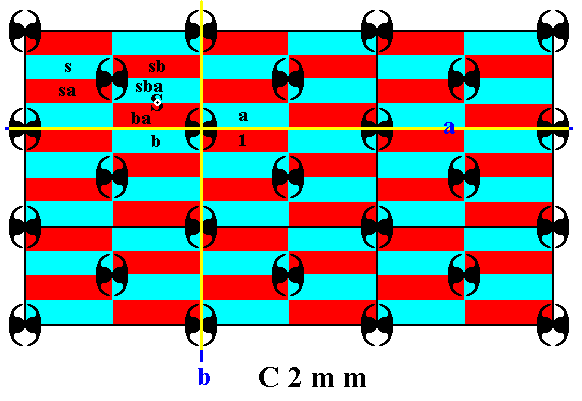Figure 16.  Generation of the group elements  ba  (by reflecting the element  a  in the mirror line  b ), and  sba, sa, sb ,  by means of the generator  s ,  which is a half-turn about the point  S .

```
```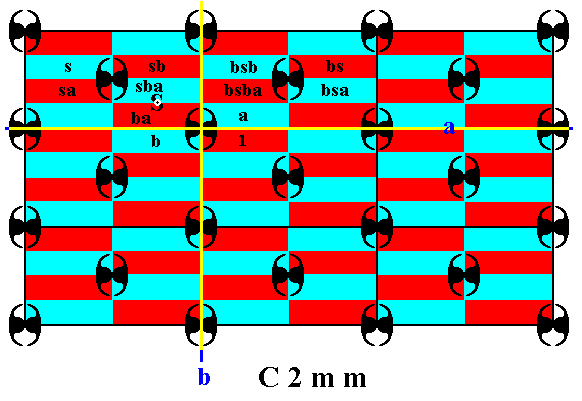Figure 17.  Generation of the group elements  bs, bsba, bsa, bsb ,  by means of the generator  b ,  which is a reflection in the mirror line  b .

```
```Figure 18.  Generation of the group elements  as, asa, asb, asba, absb, absba, abs, absa ,  by means of the generator  a ,  which is a reflection in the mirror line  a .

```
```Figure 19.  Generation of the group elements  sabsba, asabsba, bsabsba, absabsba ,  by means of the generators  s ,  a  and  b .

```
```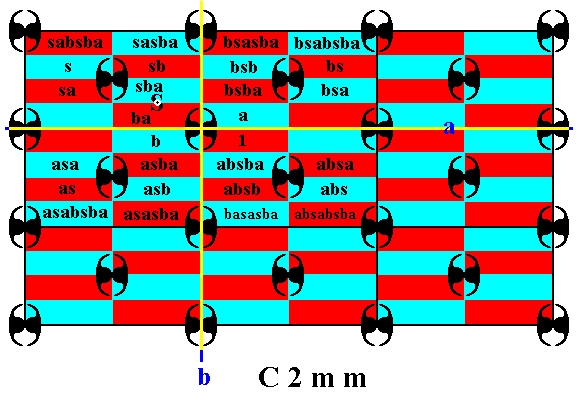Figure 20.  Generation of the group elements  sasba, bsasba, asasba, basasba ,  by means of the generators  s ,  a  and  b .

```
```Figure 21.  Generation of the group elements  sbsba, asbsba, basbsba, bsbsba ,  by means of the generators  s ,  a  and  b .

```
```
The generation process can and must be continued indefinitely. To reach the right-hand part of the displayed pattern, we can, for example subject the generated group element  absabsba  (at the lower right corner of the already filled-in area) to the transformation  s  (half-turn about the point  S ), resulting in a group element that lies to the upper left, outside the displayed part of the pattern. And if we then subject that result to the transformation  b ,  we end up at the right-hand part of the displayed pattern, and so on.
```

```
In the next document we will generate the Plane Group P4mm .

e-mail :```
```
To continue click HERE for further study of the totally dynamic and holistic nature of Reality.
```
```
back to homepage

****************